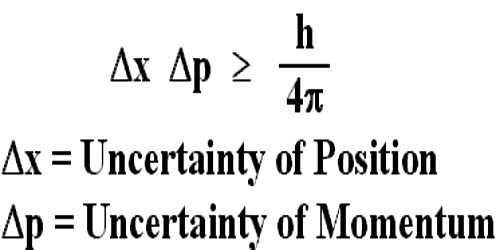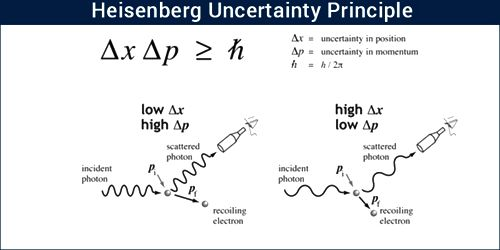Physics

# Heisenberg’s Uncertainty PrincipleHeisenberg’s Uncertainty Principle

According to De-Broglie hypothesis matter has dual characteristics – one is particle property and the other one is the wave property. When matter behaves as a particle, then according to classical mechanics its positions and momentum can be determined accurately. But when matter behaves as a wave, then its position and momentum cannot be determined accurately. This is because that wave spreads all around. So, scientist Heisenberg put forward the concept of uncertainty in the simultaneous determination of position and momentum. According to his opinion, simultaneous determination of positions and momentum of a particle accurately is impossible. That means, as the measurement of the position of a particle becomes more accurate, there will be more error in the measurement of momentum. Again, as the measurement of momentum is more accurate the measurement of the position will be less accurate. It is called the Heisenberg’s uncertainty principle.

The principle is stated below –

“If the uncertainty in the position of a particle at a particular time is Δx and uncertainty in momentum is Δp, then their product will be equal to or greater than Planck’s constant.”The principle states that it is impossible to know simultaneously the exact position and momentum of a particle. That is, the more exactly the position is determined, the less known the momentum, and vice versa.

Mathematically, Δx. Δp > h/2π; here, h = Planck’s reduced constant.

Hence, a product of the uncertainty in the determination reduced constant position and momentum of a particle in any particular direction will be equal to or greater than (h/2π). This relation is valid for energy and time of a body as well. The uncertainty of energy and time is,

ΔE.Δt > h/2π.